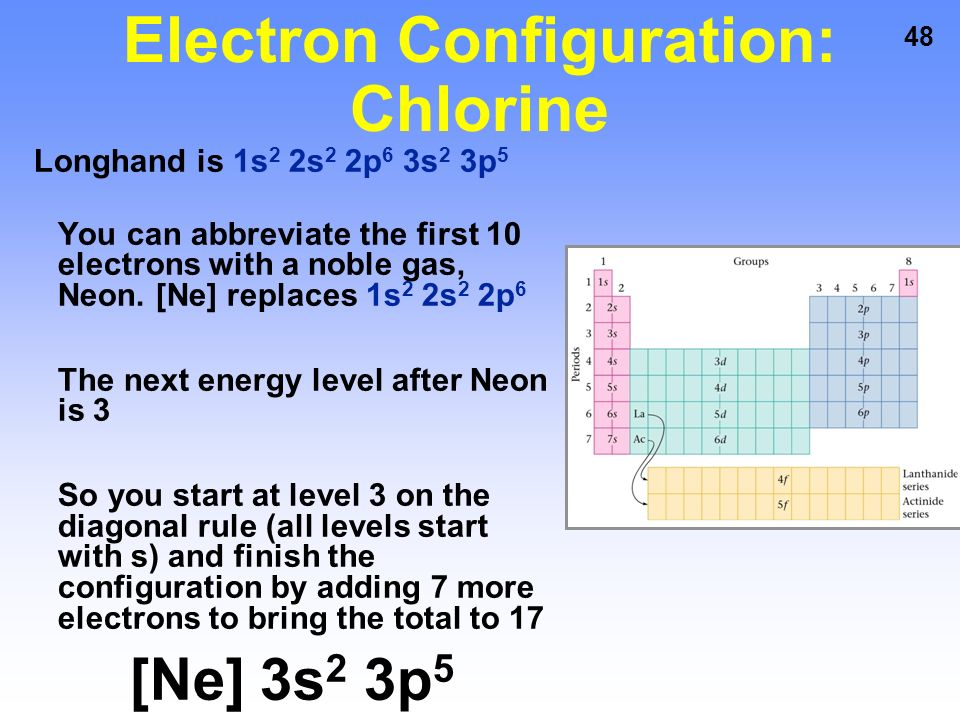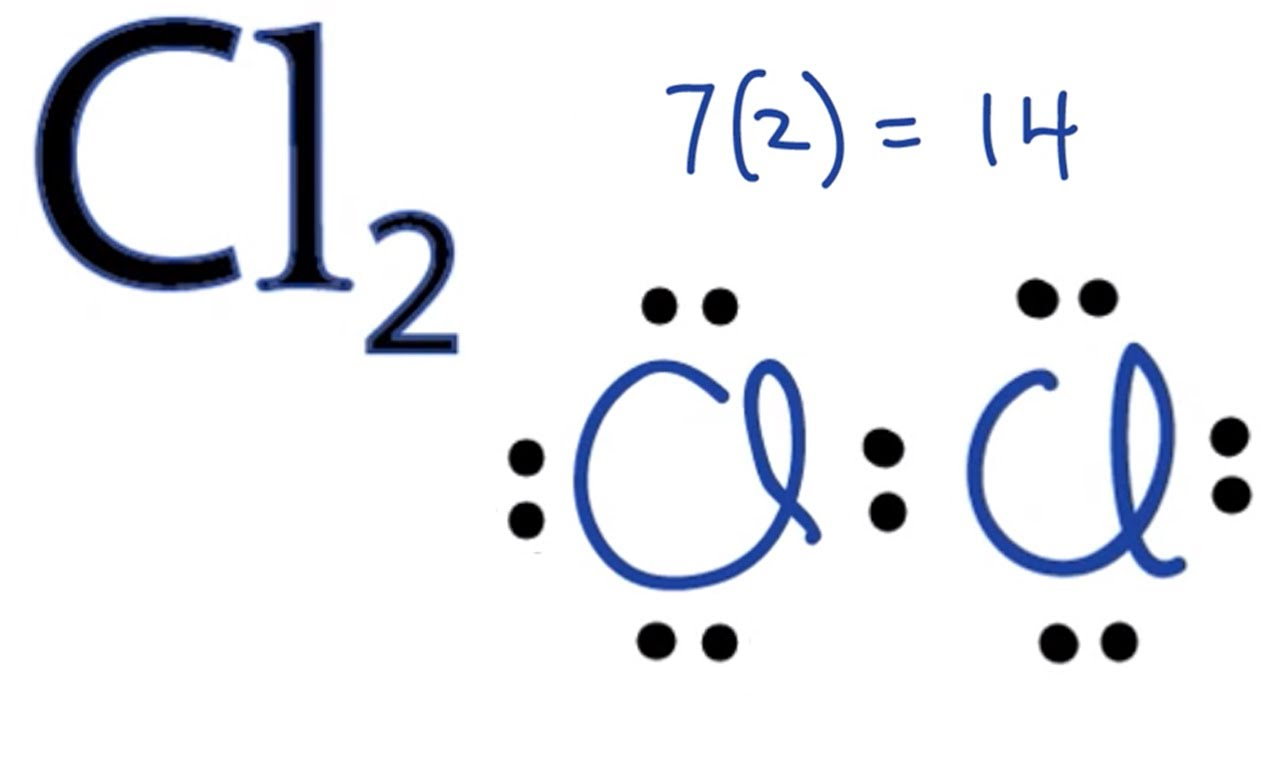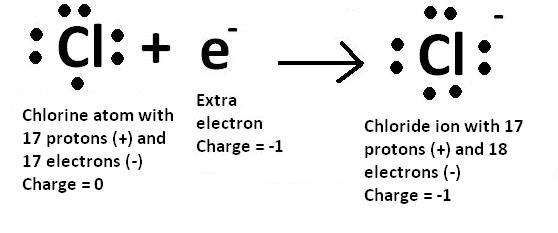# How To Find The Electron Configuration For Chlorine

For writing the Chlorine electron configuration you first need to check the number of electrons for the Chlorine (Cl) atom (there are 17 electrons in chlorine atom). When you write the configuration you will have to put all 17 electrons of the chlorine atom in the orbitals that are around the nucleus of the Chlorine atom.### Electron Dot Structure of Chlorine

The Lewis dot structure for Cl( Chlorine). Note that Chlorine is in Group of 17 (which is sometimes called Group VII or 7A). It will have 7 valence electrons Since it is in Group 7. You can see the electron dot structure of chlorine in the image below;### Chlorine Number of Valence Electrons

The atomic number of chlorine is 17. It is the number of protons that are present in the nucleus of an atom. All the atoms of chlorine have the same atomic number. In every atom that is stable, the number of electrons and the number of protons are equal. Similarly, the number of electrons and protons in chlorine atom. This means, that the number of electrons present in the chlorine atom is 17.## What is Electron For Chlorine

Since the atomic number of the chlorine is 17 so the number of electron in the chlorine is 17 You can also check out the image below;

## Electronic Configuration For Chlorine Ion

You can see the electron configuration of a chlorine atom (Cl) below:

1s22s22p63s23p5

Cl (-) is as follow;

1s22s22p63s23p6